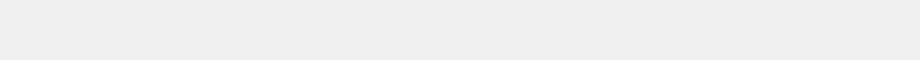API Details
Random Real Numbers
NousMind, NousCrypt, and NousRandom are trademarks of Scott Michael Doctor
Patents Pending
The Random Real Numbers API sends random 64-bit floating point resolution numbers. The actual resolution is 52 bits accounting for the exponent and sign bits. The default format is normal floating point decimal with 16 digits and a decimal point. If scaling to very large or very small values, you can choose to have the number sent in scientific notation. The following are the API commands for this process:

flt -- This is the default. This is the process ID. Each process has an ID to tell the API what to generate. flt specifies that you want random floating point numbers.

min=n -- Sets the minimum value for scaling the numbers. Default: -1.0
n
is any valid floating point number specified by an integer, floating point decimal, or scientific notation number. min and max cannot be equal.  You must set a value for max if setting a value for min.

max=n -- Sets the maximum value for scaling the numbers. Default: 1.0
n
is any valid floating point number specified by an integer, floating point decimal, or scientific notation number.  min and max cannot be equal.

If you set max without setting min, the scaling will be set to +/- the value of max. For example, if max=3.0 without specifying a value for min, then min will be set by the API to -3.0.

The value of max should be the greater than the value of min. However, the API always checks both values and if it finds that max is less than min, then the API will swap their values.

cnt=d -- Sets the total count of numbers to send. Default: 1
d is a positive integer greater than zero. A negative value for d returns an error. The value of d can be a floating point number. However, only the integer value of the number is used. For example, a value of 4.9 will set the value of cnt to 4. The API does not round the value, simply truncates the fractional part. The maximum count of numbers per request is 1,048,576 which is 2^20.

Note that cnt is the Total count of numbers. For example, if you want 20 rows of numbers with 4 columns of numbers in each row, that is a total of 80 numbers, so you would set cnt=80.

cols=d -- Sets the number of columns per row. Default: 1
d is a positive integer greater or equal to zero. A negative value for d returns an error. The value of d can be a floating point number. However, only the integer value of the number is used. For example, a value of 4.9 will set the value of cols to 4. The API does not round the value, simply truncates the fractional part.

If cols is set to 0, then all of the numbers will be sent in a single line.
If cols is greater than the value of cnt, then cols is set by the API to the value of cnt.

scioff -- Turns off scientific notation. This is the default.
All numbers are sent as normal floating point decimal.

sciall -- All numbers sent in scientific notation.

scithresh -- Sends the value in scientific notation only if it exceeds the thresholds.
If the absolute value is less than scimin, or greater/equal to scimax, then the value is sent in scientific notation, else it is sent as normal floating point decimal.

scimin=n -- Used with scithresh. The lower threshold. Default: 0.0001
n
is any valid floating point number specified by an integer, floating point decimal, or scientific notation number. You can specify a negative number, however, the API uses the absolute value of n.

If the value is less than scimin, and scithresh is specified, then it is sent in scientific notation.

scimax=n -- Used with scithresh. The upper threshold. Default: 1000000000.0   (1e9)
n is any valid floating point number specified by an integer, floating point decimal, or scientific notation number. You can specify a negative number, however, the API uses the absolute value of the n.

If the value is greater or equal to scimax, and scithresh is specified, then it is sent in scientific notation.

The following are common formatting options. Full details are on the Results Formatting page.

nohtml -- disables sending HTML tags. The tags are used for proper display in a web browser. HTML tags are not used if the numbers are sent as a text file.

astextfile -- Send the numbers as a text file. If the total count of numbers sent exceeds 16384, then the numbers will always be sent as a file.

csv -- Separate columns of numbers with a single space character.

csvq -- Same as csv with each number surrounded by the double quote character.

json -- Sends the numbers as a JSON array. See the Results Formatting page for details.

jsonq -- Same as json with each number surrounded by the double quote character.